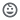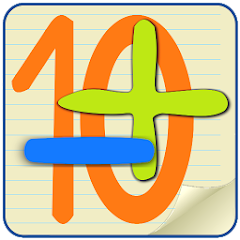# Mathematics 10

10+Everyone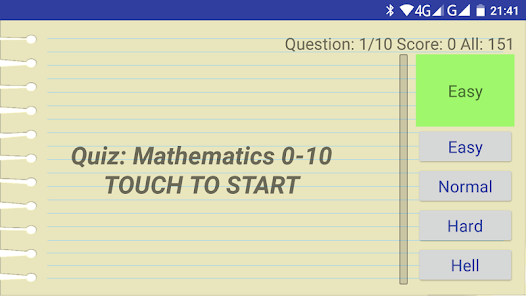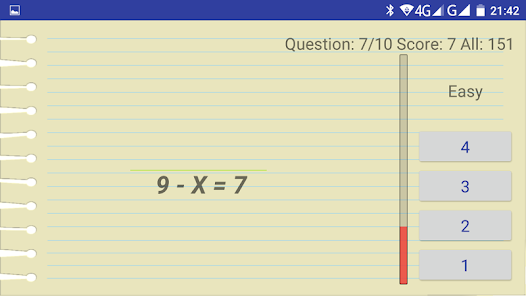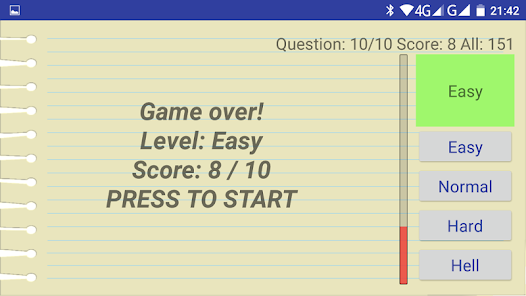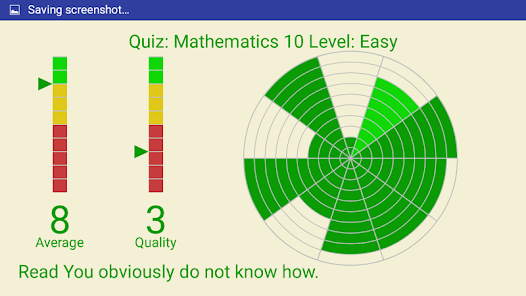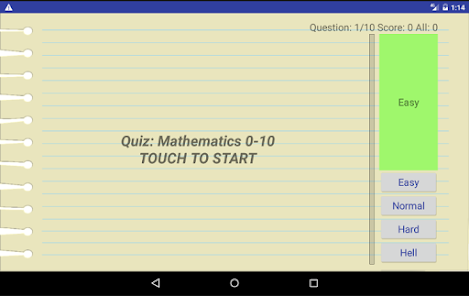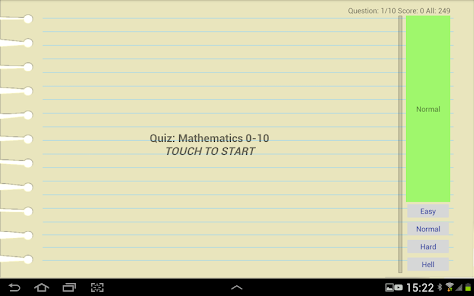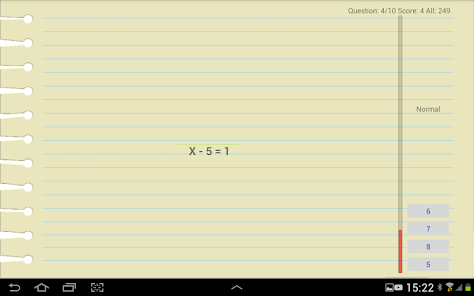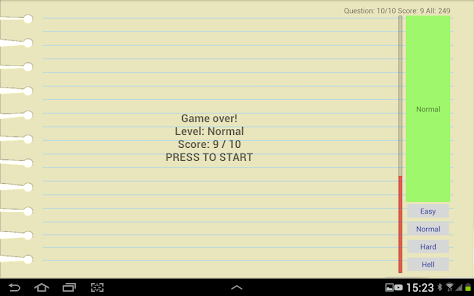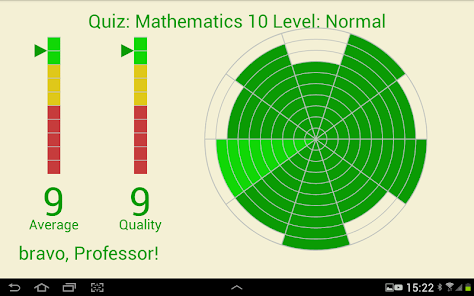Mathematics quiz. Addition, substraction, compare 0-10. Kindergarten.
Teaching test in mathematics for primary school pupils (1st grade).
Add up to 10, subtract to 10, compare numbers to 10, solve equations up to 10.
Four levels of complexity, extended statistics for each level.
Assignments of the form: 4 + X = 8, 5 + 3 = X, 9-X = 5, X-1 = 8, X <4, 7> X.
The relationship between the numbers (greater, less, equal).
Addition and subtraction operations, + and - signs. Amount, difference.
The addition table is within 10.
Minuend. Subtrahend. Difference. Finding an unknown term. Subtraction 6, 7, 8 and 9.

In the math test for class 1, the time limit for each answer is used, the more complex the level the less time is given to choose the correct answer.
In appendix 352 of the question, when starting from them, 10 questions are selected in random order.
If all the answers are correct then you can score 10 points. Points are deducted for the wrong answer and for exceeding the time limit.
Updated on
May 26, 2017

## Data safety

Safety starts with understanding how developers collect and share your data. Data privacy and security practices may vary based on your use, region, and age. The developer provided this information and may update it over time.No data shared with third parties